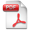## Archive for mayo, 2014

### THE FERMAT’S PROBLEM

// mayo 23rd, 2014 // No Comments » // Science

This conjecture1 asserts that the diophantine equation: zp = xp + yp (1) only admits integer and positive solutions for p < 3 , as already asserted Pierre Simon de FERMAT (1601 – 1665) in an annotation to the Arithmetic of DIOPHANTUS OF ALEXANDRIA (probably s. II D.C.). For p = 2 we have the famous “Pythagorean equation” that has infinite solutions, the most known is the “Egyptian triangle”: 32 + 42 = 52 . EULER demonstrated the impossibility of (1) for p = 3 and p = 4 ; DIRICHLET for p = 5 ; LAMÉ for p = 7 ; LEGENDRE for p = 14 ; KUMMER studied it for several categories of numbers.PDF Document